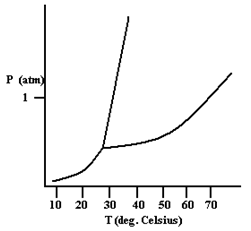# Problem: Based on the phase diagram shown below, how will the melting point of the substance change if the pressure is increased above 1 atm? A)      The melting point will decrease. B)      The melting point will remain the same. C)      The melting point will increase. D)      The substance will not melt at pressures of 1 atm and above; instead, the solid sublimes to form the gas phase.

###### FREE Expert Solution
82% (345 ratings)
###### Problem Details

Based on the phase diagram shown below, how will the melting point of the substance change if the pressure is increased above 1 atm?

A)      The melting point will decrease.

B)      The melting point will remain the same.

C)      The melting point will increase.

D)      The substance will not melt at pressures of 1 atm and above; instead, the solid sublimes to form the gas phase.What scientific concept do you need to know in order to solve this problem?

Our tutors have indicated that to solve this problem you will need to apply the Phase Diagram concept. You can view video lessons to learn Phase Diagram. Or if you need more Phase Diagram practice, you can also practice Phase Diagram practice problems.

What is the difficulty of this problem?

Our tutors rated the difficulty ofBased on the phase diagram shown below, how will the melting...as low difficulty.

How long does this problem take to solve?

Our expert Chemistry tutor, Ellen took 1 minute and 41 seconds to solve this problem. You can follow their steps in the video explanation above.

What professor is this problem relevant for?

Based on our data, we think this problem is relevant for Professor Tang's class at USF.